## [论文阅读笔记]DeepFool: a simple and accurate method to fool deep neural networks-程序员宅基地

#### DeepFool: a simple and accurate method to fool deep neural networks(2016 CVPR)

• 这是一种untargeted attak
• 该算法是通过寻求当前的点在高维空间中离所有非真实类的决策边界中最近的一个，来作为攻击后的label
• 需要注意的是，该算法是一种贪心算法，并不能保证收敛到(1)中的最优扰动。但是，作者在实践中观察到，该算法产生的扰动非常小，可以认为是最小扰动的很好的近似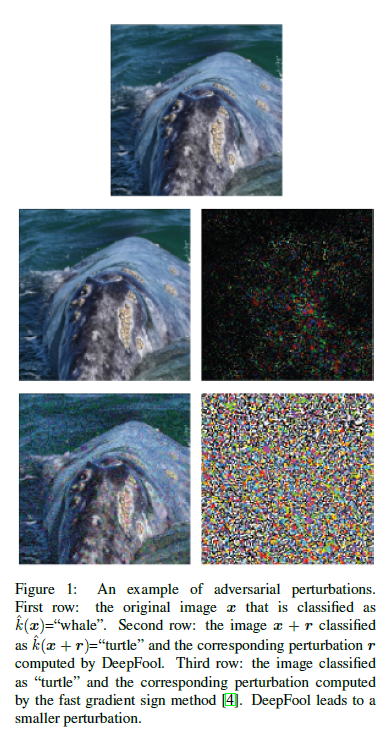Contribute：

• 提出了一种简单、准确的方法来计算比较不对分类器对对抗扰动的鲁棒性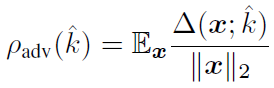其中 ∇ ( x ; k ^ ) \nabla (x; \hat{k})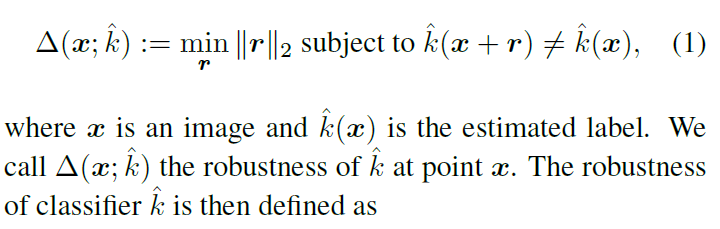• 做了广泛的实验比较从而得出
a) 我们的方法比现有的方法更可靠、更有效地计算了对抗性扰动。
b) 发现用对抗性的例子增加训练数据可以显著增强对对抗性扰动的鲁棒性。

• 作者证明，使用不精确的方法来计算对抗性扰动，可能导致对鲁棒性的不同结论，有时还会产生误导。因此，作者的方法提供了一个更好的理解这个有趣的现象及其影响因素。

DeepFool for binary classifiers：
f f 是一个线性二分类分类器： f ( x ) = w T x + b f(x)=w^Tx+b 。如下图，为了使得扰动达到最小，最小的方向就是垂直于分类面的方向。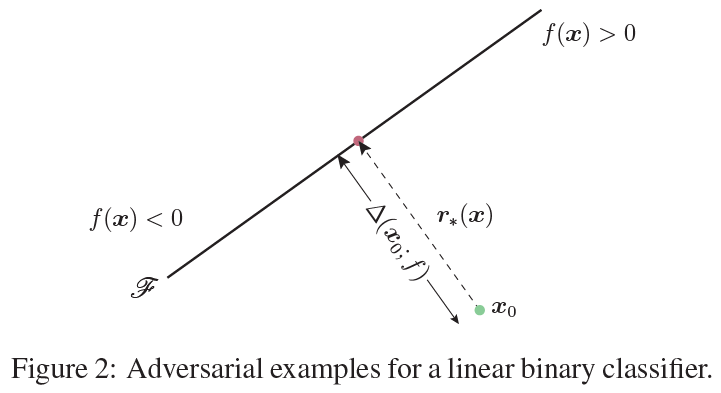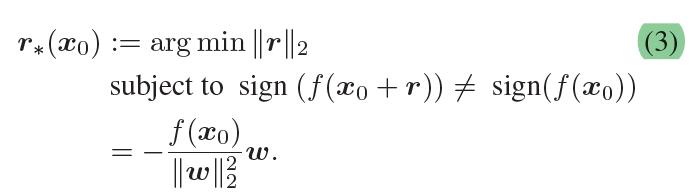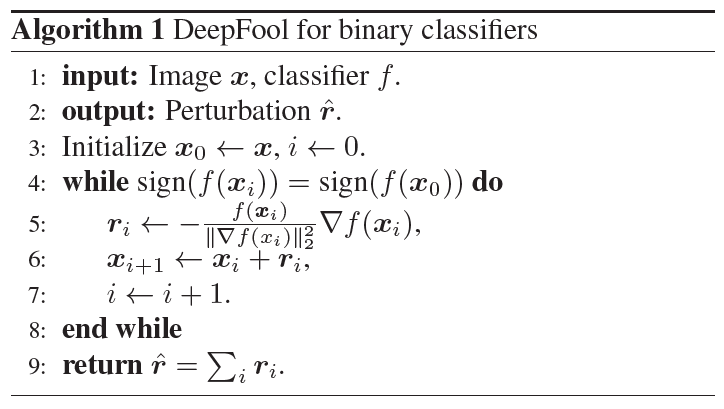No！
f ( x 0 ) ∣ ∣ w ∣ ∣ 2 2 w ⇒ f ( x 0 ) ∣ ∣ w ∣ ∣ 2 w ∣ ∣ w ∣ ∣ 2 \frac{f(x_0)}{||w||_2^2} w \Rightarrow \frac{f(x_0)}{||w||_2} \frac{w}{||w||_2}

f ( x 0 ) ∣ ∣ w ∣ ∣ 2 \frac{f(x_0)}{||w||_2}

w ∣ ∣ w ∣ ∣ 2 \frac{w}{||w||_2}

DeepFool for multiclass classifiers：

• 本文采用的多分类器方案是one-vs-all
• 这里依然考虑的线性分类器
• 分类的结果的选择 k ^ ( x ) = arg ⁡ max ⁡ k f k ( x ) \hat{k}(x)= \underset{k}{\arg \max} f_k (x) 其中 f k ( x ) f_k(x) f ( x ) f(x) k t h k_{th} 的输出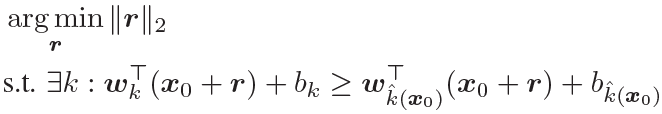k ^ ( x 0 ) = 4 \hat{k}(x_0)=4 而言，假设其决策边界为下图，为了用最小的扰动来达到攻击效果，从图中我们可以选择往第3个边界移动。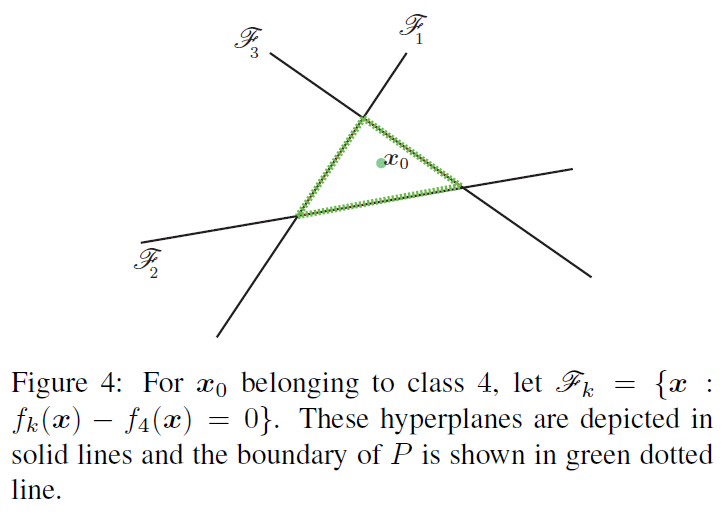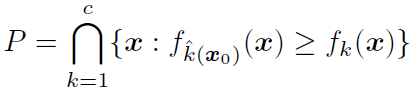l ^ ( x 0 ) \hat{l}(x_0) 为距离P的边界最近的超平面，其可以通过下式进行计算。有点不明白为什么要通过以下公式计算最近的超平面，而不是直接用点到平面的距离公式？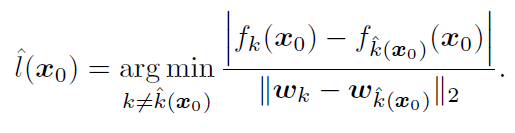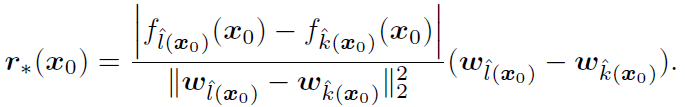DeepFool for General classifiers：

• 此时可以是更一般的非线性的分类器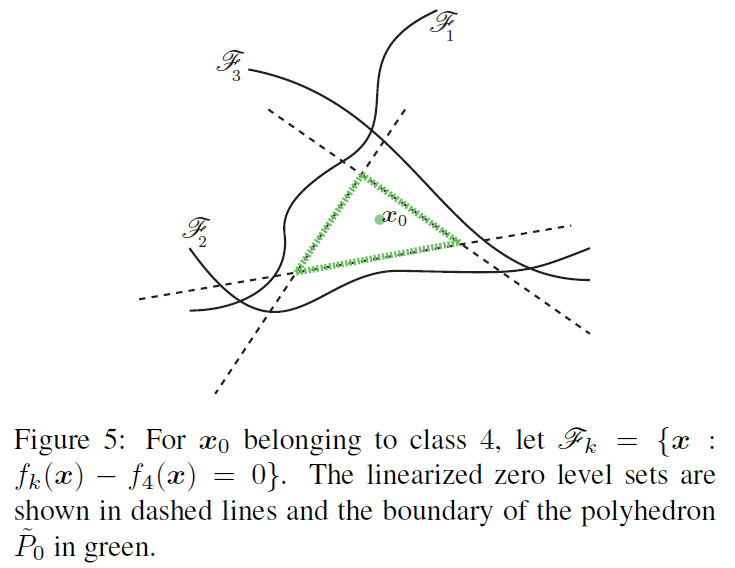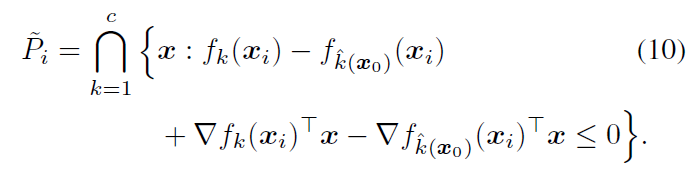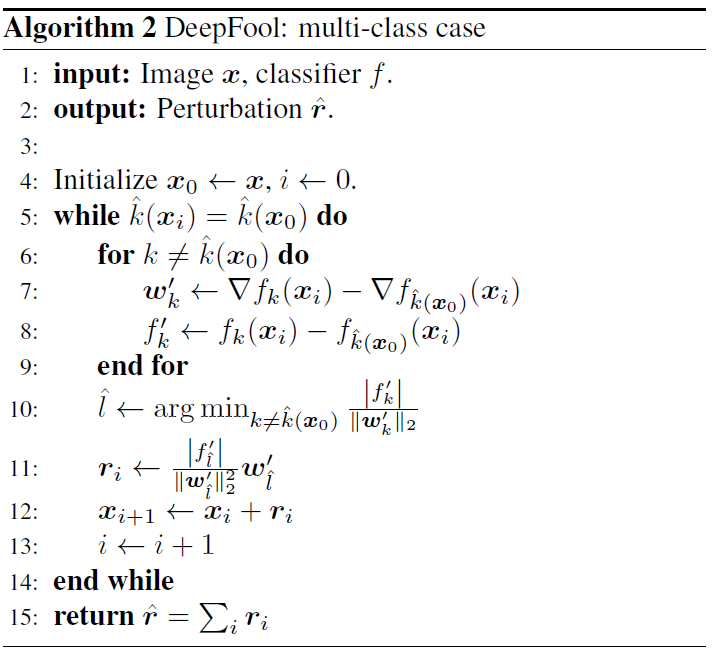Conclusion:

• 本文提出了一种新的算法DeepFool
• 本文基于分类器的iterative linearization来产生较小的扰动并产生非常有效的攻击效果
• 本文在三个数据集和八个分类器上提供了大量的实验证据，表明了该方法相对于现有的计算对抗性扰动的方法的优越性，以及该方法的有效性。